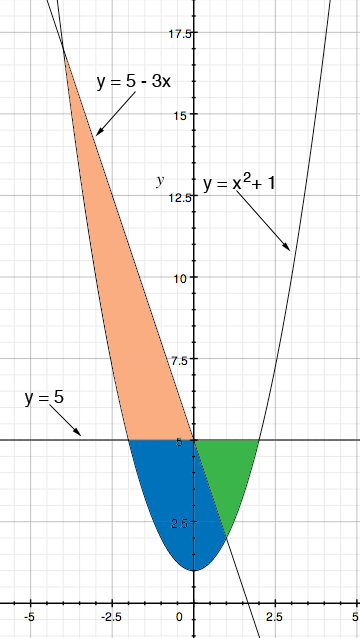SEARCH HOMEMath Central Quandaries & QueriesQuestion from ted, a student: Consider the region bounded by y=x^2 + 1, y=5-3x and y=5. Sketch and shade the given region; then set up but don't evaluate the integrals to find the following: a) The volume of the solid generated by rotating the region about the line y=5 b) the volume of the solid generated by rotating the region about the y-axisHi Ted,

I sketched the three curves and I see 3 regions that are bounded by the curves.I think the problem should say "Consider the region in the first quadrant bounded by..." That would make it the region shaded green in my diagram.

Now look at my response to a similar question.

Does that help? If you need further assistance write back tell us what you have done and we will try to help.

PennyMath Central is supported by the University of Regina and The Pacific Institute for the Mathematical Sciences.